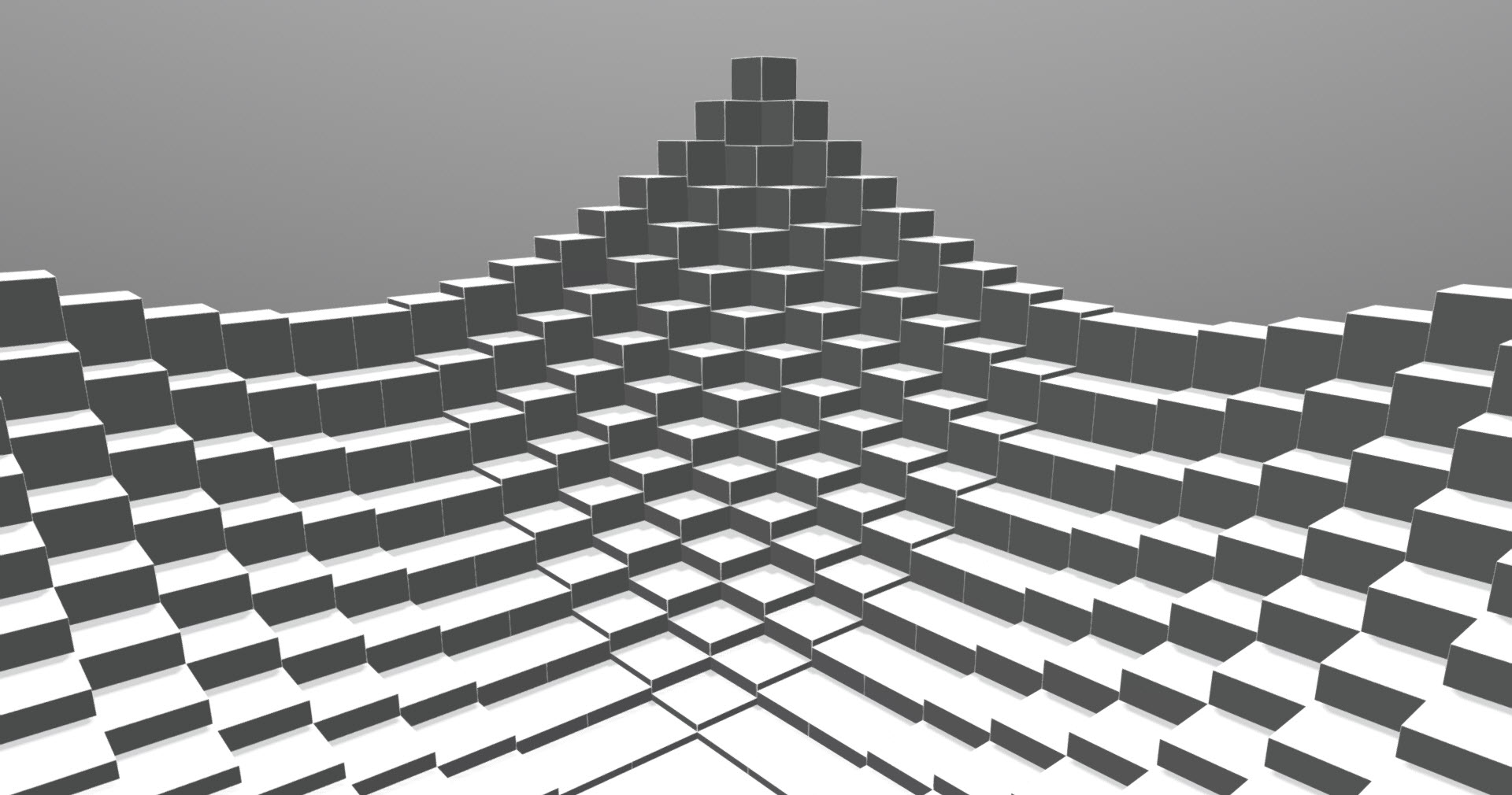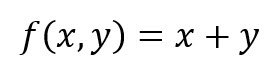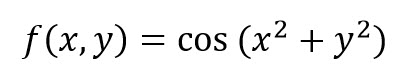Scripting geometry (as described in Chapter 7) can make things look very interesting (and quite beautiful) with a rather small amount of actual code. You can see this in the patterned panel example or in the pulled brick post. Both of those examples use a combination of sine and cosine functions to create a “wavy” pattern that nicely undulates shapes and geometry. There are other functions, however, some of which you likely remember from algebra and trigonometry classes. But even without delving too deeply into this, I thought it would be useful to have a reference for some interesting functions that may be of use.

The interactive examples shown below use the following grid of 1 inch cubes in SketchUp that were simply scaled vertically according to the lines of code that are included at the bottom of this post. Obviously you can use this approach for other purposes and in other ways, too, but this is a good way to explain the formulas’ behavior.

Also, as you can see in the code snippet, including various numeric values (by dividing or multiplying) allows you to stretch and scale the functions to your needs. Vary those as needed and don’t be shy to try things out. There’s always the undo button!

Finally, if you give the code snippet below a try then it is easy to combine functions by replacing `f5` in the following line with e.g. `f1 + f3`.

`t = Geom::Transformation.scaling( 1, 1, f5 )`

Have fun experimenting with these…

## Linear in x and y

These functions follow a pattern like:## Double parabola:

These functions follow a pattern like:## Diagonal sine wave

These functions follow a pattern like:## Waves in x and y

These functions follow a pattern like: